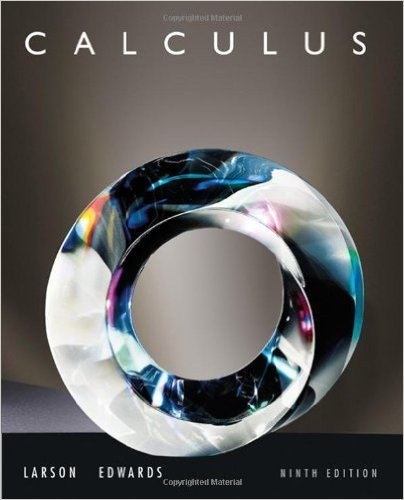×
×

# Solutions for Chapter 10: Conics, Parametric Equations, and Polar Coordinates## Full solutions for Calculus | 9th Edition

ISBN: 9780547167022Solutions for Chapter 10: Conics, Parametric Equations, and Polar Coordinates

Solutions for Chapter 10
4 5 0 261 Reviews
22
3
##### ISBN: 9780547167022

This expansive textbook survival guide covers the following chapters and their solutions. Chapter 10: Conics, Parametric Equations, and Polar Coordinates includes 127 full step-by-step solutions. Since 127 problems in chapter 10: Conics, Parametric Equations, and Polar Coordinates have been answered, more than 61809 students have viewed full step-by-step solutions from this chapter. This textbook survival guide was created for the textbook: Calculus , edition: 9. Calculus was written by and is associated to the ISBN: 9780547167022.

Key Calculus Terms and definitions covered in this textbook
• Absolute minimum

A value ƒ(c) is an absolute minimum value of ƒ if ƒ(c) ? ƒ(x)for all x in the domain of ƒ.

• Additive identity for the complex numbers

0 + 0i is the complex number zero

• Component form of a vector

If a vector’s representative in standard position has a terminal point (a,b) (or (a, b, c)) , then (a,b) (or (a, b, c)) is the component form of the vector, and a and b are the horizontal and vertical components of the vector (or a, b, and c are the x-, y-, and z-components of the vector, respectively)

• Constant term

See Polynomial function

• Domain of validity of an identity

The set of values of the variable for which both sides of the identity are defined

• End behavior

The behavior of a graph of a function as.

• Extracting square roots

A method for solving equations in the form x 2 = k.

• Histogram

A graph that visually represents the information in a frequency table using rectangular areas proportional to the frequencies.

• Implicitly defined function

A function that is a subset of a relation defined by an equation in x and y.

• Increasing on an interval

A function ƒ is increasing on an interval I if, for any two points in I, a positive change in x results in a positive change in.

• Inductive step

See Mathematical induction.

• Inverse composition rule

The composition of a one-toone function with its inverse results in the identity function.

• Limit at infinity

limx: qƒ1x2 = L means that ƒ1x2 gets arbitrarily close to L as x gets arbitrarily large; lim x:- q ƒ1x2 means that gets arbitrarily close to L as gets arbitrarily large

• Logarithmic re-expression of data

Transformation of a data set involving the natural logarithm: exponential regression, natural logarithmic regression, power regression

• Matrix, m x n

A rectangular array of m rows and n columns of real numbers

• Position vector of the point (a, b)

The vector <a,b>.

• Quotient of complex numbers

a + bi c + di = ac + bd c2 + d2 + bc - ad c2 + d2 i

• Reference angle

See Reference triangle

• Rigid transformation

A transformation that leaves the basic shape of a graph unchanged.

• Sample survey

A process for gathering data from a subset of a population, usually through direct questioning.

×